• Slides: 18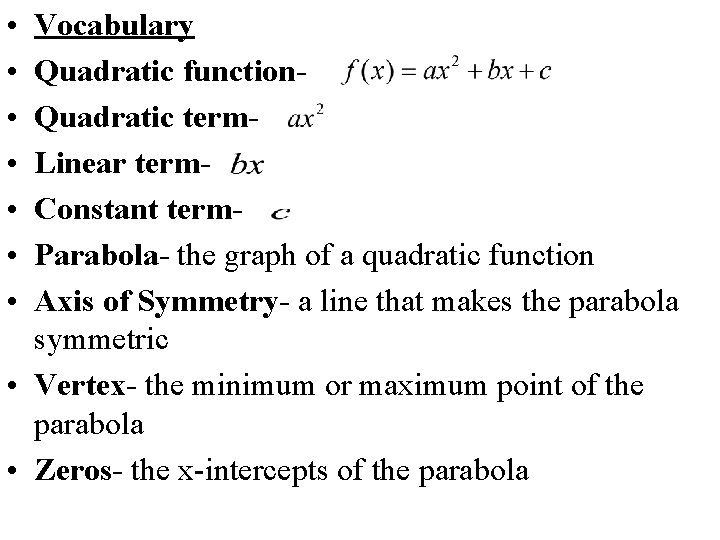• • Vocabulary Quadratic function. Quadratic term. Linear term. Constant term. Parabola- the graph of a quadratic function Axis of Symmetry- a line that makes the parabola symmetric • Vertex- the minimum or maximum point of the parabola • Zeros- the x-intercepts of the parabola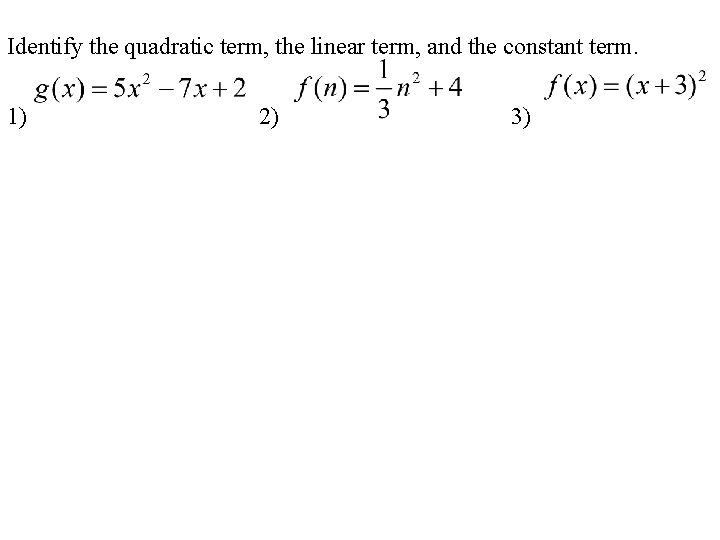Identify the quadratic term, the linear term, and the constant term. 1) 2) 3)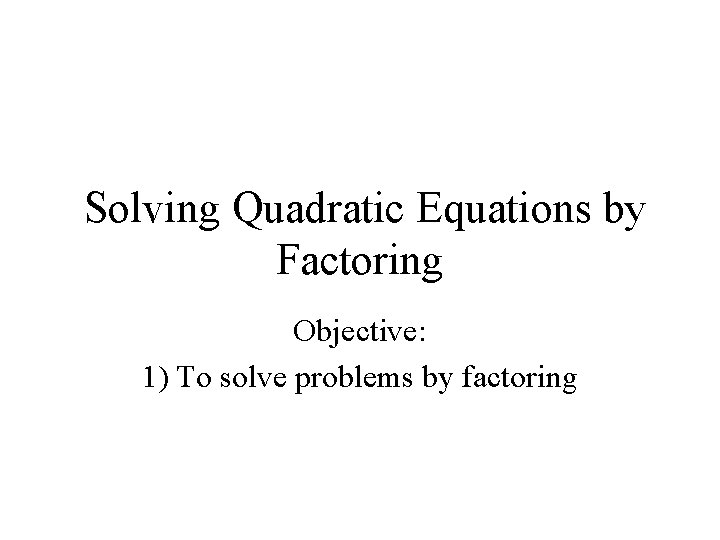Solving Quadratic Equations by Factoring Objective: 1) To solve problems by factoring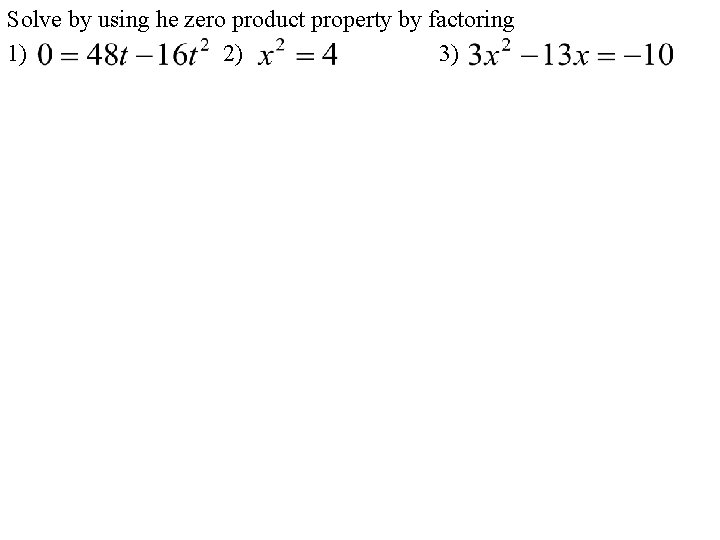Solve by using he zero product property by factoring 1) 2) 3)The Quadratic Formula and the Discriminant Objective: 1) To solve quadratic equations by using the quadratic formula 2) To use the discriminant to determine the nature of the roots of quadratic equations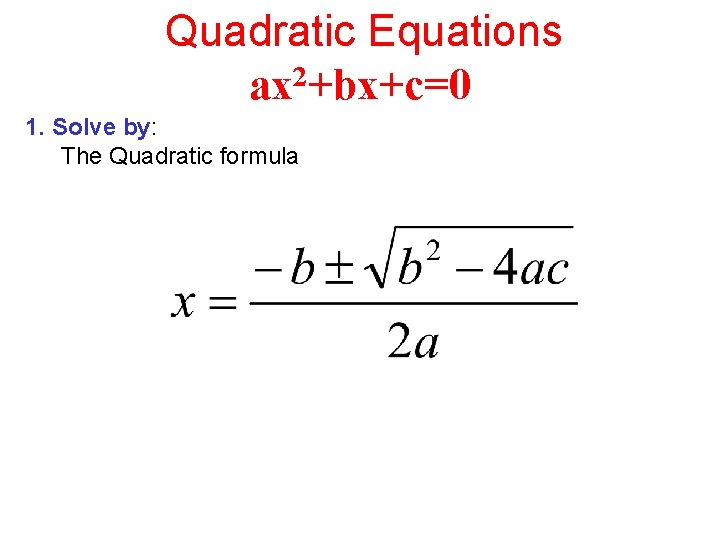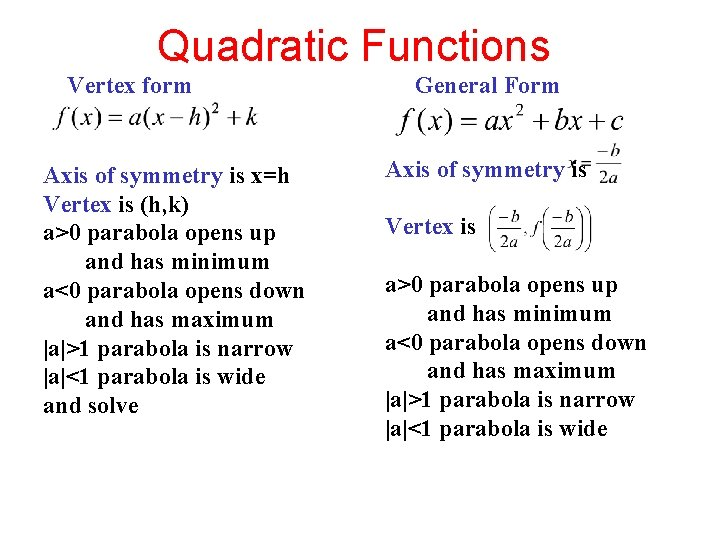Quadratic Functions Vertex form Axis of symmetry is x=h Vertex is (h, k) a>0 parabola opens up and has minimum a<0 parabola opens down and has maximum |a|>1 parabola is narrow |a|<1 parabola is wide and solve General Form Axis of symmetry is Vertex is a>0 parabola opens up and has minimum a<0 parabola opens down and has maximum |a|>1 parabola is narrow |a|<1 parabola is wide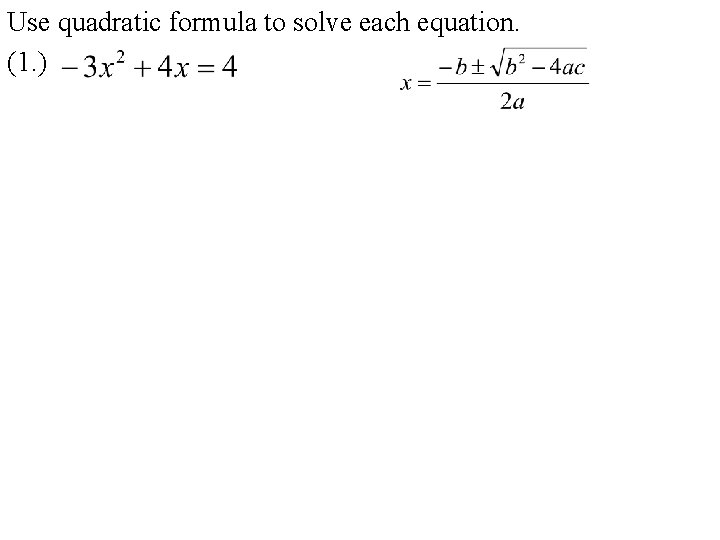Use quadratic formula to solve each equation. (1. )Use quadratic formula to solve each equation. (2. )Examples Value of Discriminant a Perfect Square? Nature of Roots 1 Greater than zero Yes 2 real, rational #’s 2 Greater than zero Yes 2 real, Irrational #’s 3 Less than zero na 2 imaginary #’s 4 Zero na 1 real #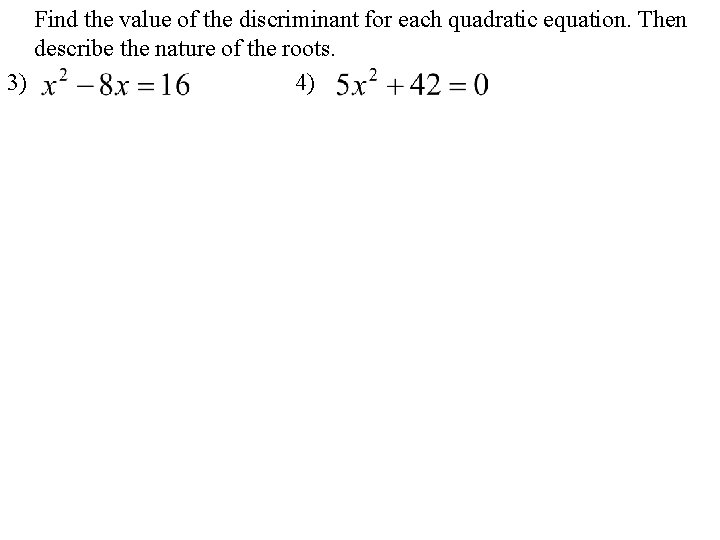Find the value of the discriminant for each quadratic equation. Then describe the nature of the roots. 3) 4)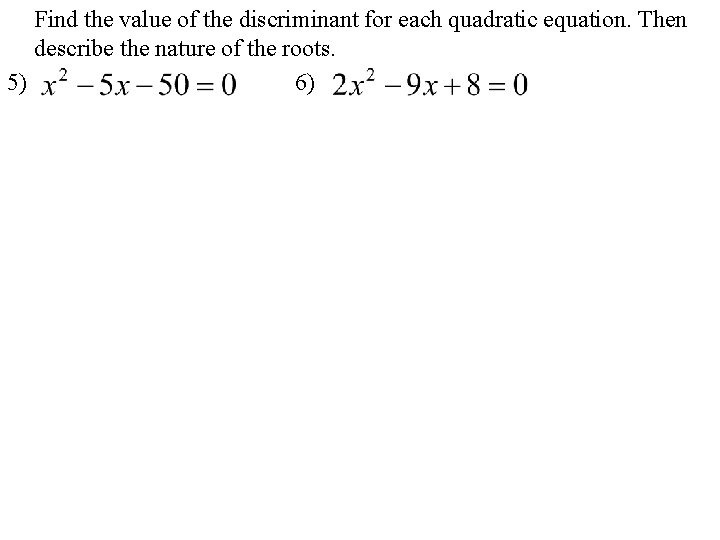Find the value of the discriminant for each quadratic equation. Then describe the nature of the roots. 5) 6)Vertex to Standard Form Notes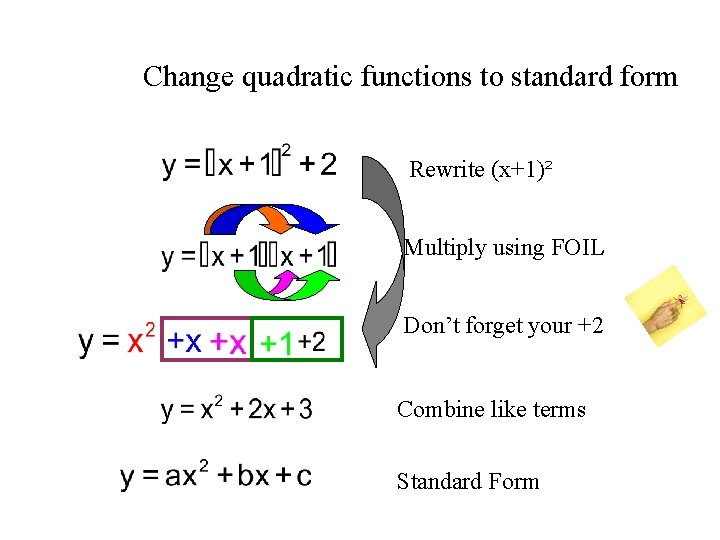Change quadratic functions to standard form Rewrite (x+1)² Multiply using FOIL Don’t forget your +2 Combine like terms Standard Form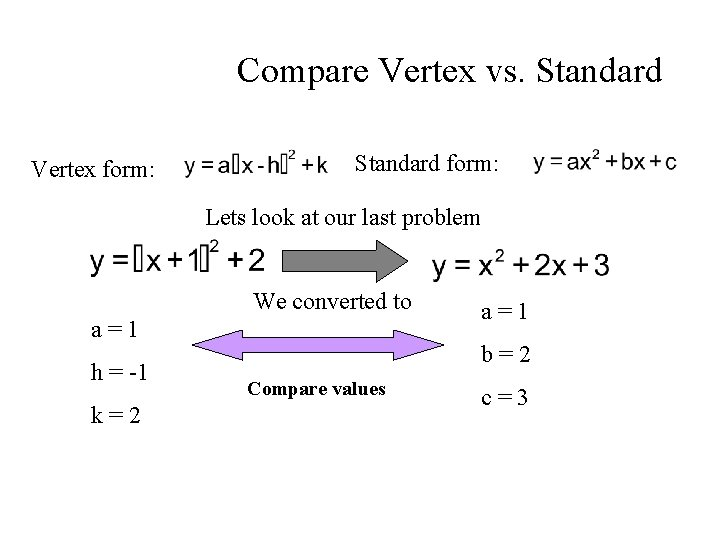Compare Vertex vs. Standard Vertex form: Standard form: Lets look at our last problem We converted to a=1 h = -1 k=2 a=1 b=2 Compare values c=3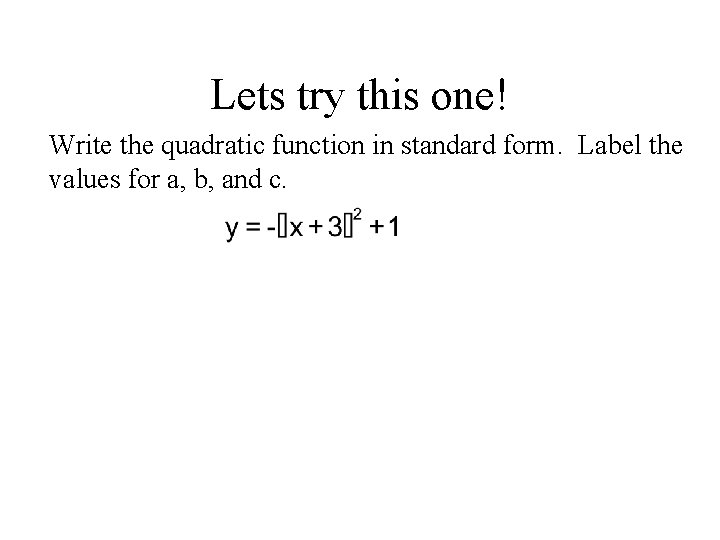Lets try this one! Write the quadratic function in standard form. Label the values for a, b, and c.Your Turn Write the quadratic function in standard form. Label the values for a, b, and c.